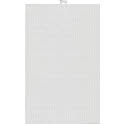plastic canvasi need to know what the hole number count is for the 13.5 by 21.5 how many holes across by how many down
Posted by: trickydicky on 12/20/16
Replies:Hello,

It's a 7 mesh canvas so that means there are 7 holes per inch. If the total size of the sheet in inches is 13.5 x 21.5 just multiply those numbers by 7 and that will give you your total hole amounts for width and height. 13.5 x 7 = 94.5 and 21.5 x 7 = 150.5.

~Kriti @ EPC
by: Kristi @ EPC on 12/21/16